# GRE Math : How to find the length of the side of an equilateral triangle

## Example Questions

### Example Question #1 : Equilateral Triangles

What is the length of a side of an equilateral triangle if the area is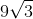?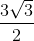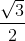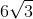Explanation:

The area of an equilateral triangle is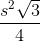.

So let's set-up an equation to solve for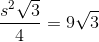Cross multiply.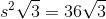The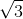cancels out and we get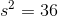.

Then take square root on both sides and we getas the final answer.

### Example Question #2 : Equilateral Triangles

If the height of the equilateral triangle is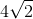, then what is the length of a side of an equilateral triangle?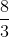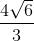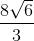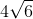Explanation:

By having a height in an equilateral triangle, the angle is bisected therefore creating two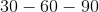triangles.

The height is opposite the angle. We can set-up a proportion.

Side oppositeisand the side of equilateral triangle which is oppositeis.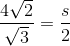Cross multiply.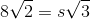Divide both sides by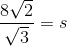Multiply top and bottom byto get rid of the radical.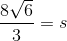Tired of practice problems?

Try live online GRE Math prep today.